|

# 一站家装,设计先行,壹号馨家家装体验馆首秀京城

6月3日，经过近一年的精心筹备，京城规模最大的一站式全透明家装服务体验品牌壹号馨家家装体验馆在北京东五环红庄创新园盛大开业。中国建筑设计院有限公司总建筑师孟建国，中国建筑装饰协会信息与科技委员会秘书长孙晓勇出席开业仪式，并与壹号馨家全国合作供应商品牌代表，京城各大主流媒体，以及到场的数百装修用户，共同见证了壹号馨家家装体验馆这一京城全新家装服务旗舰品牌的精彩亮相。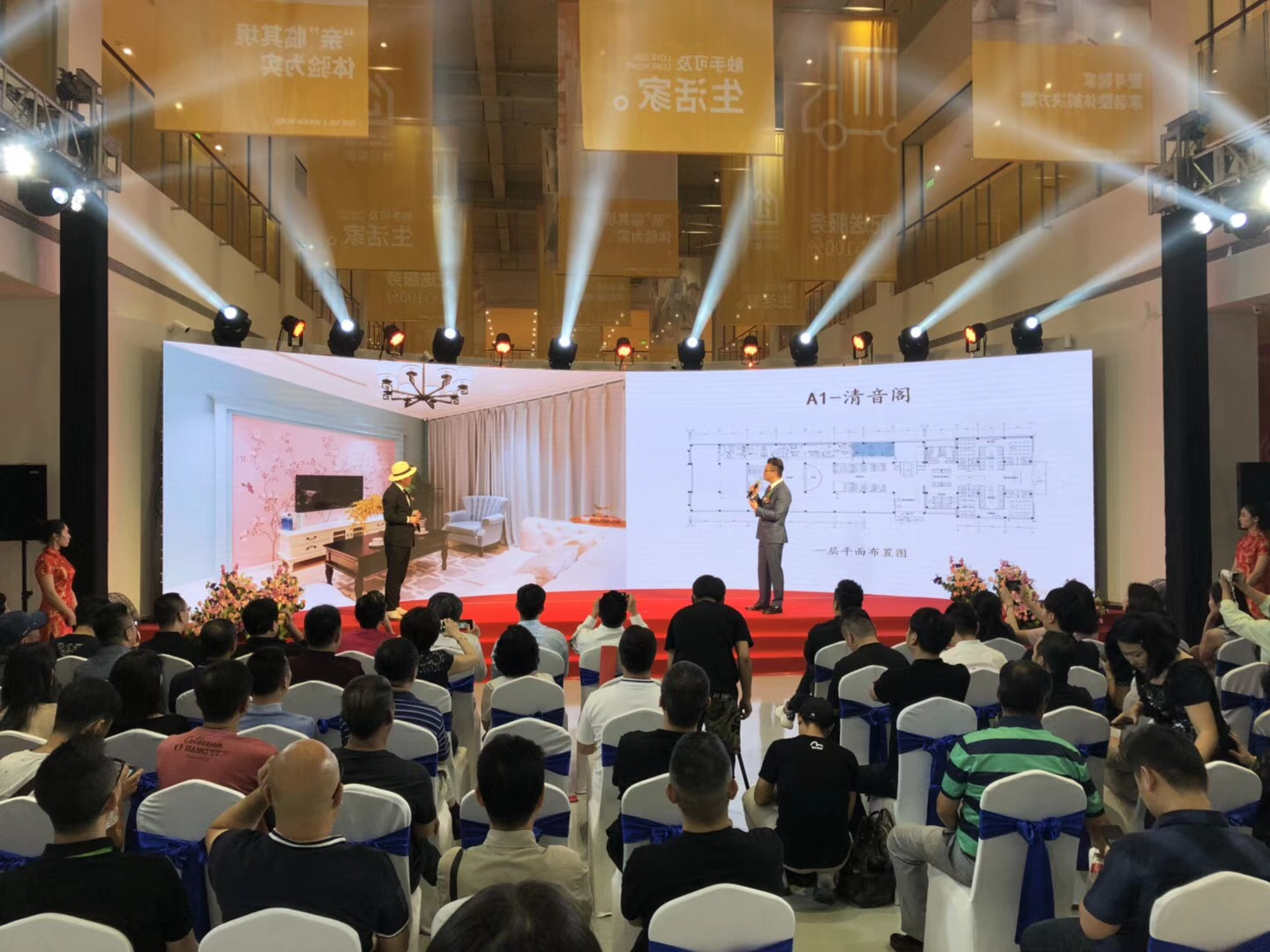据悉，壹号馨家家装体验馆定位于一站式整体家装服务，整个展厅面积近6000平米，为京城消费者带来一价全包、高性价比的家装体验。在国内知名设计师吴巍的带领下，壹号馨家的设计师团队在进行了大范围的市场调研与创意构思后，在馆内为广大消费者打造了12套实景样板间，风格包括清新北欧、现代工业、温婉简约、新中式、日式等，消费者亲临展厅即可亲身感受到超大规模、超震撼的全新家装感官新体验。

家装新锐耀目起航以产品化改变传统家装模式

上午10点30分，开业仪式在喜庆的礼炮声中拉开了帷幕。中国建筑设计院有限公司总建筑师孟建国首先致辞，高度评价了壹号馨家的服务理念。孟建国认为，家对于每个人来说都不只是一栋单纯的房子，更是一种对待生活的态度，只有高品质的家居才能带来充满幸福感的生活。现在越来越多的人愿意在家装中融入设计，同时追求更高性价比的家装服务。壹号馨家正是将这两者进行了最完美的结合，让消费者不仅可以享受到质优价优的家装服务，更能拥有优雅、舒适的居家空间，住的更加精彩！

中国建筑装饰协会信息与科技委员会秘书长孙晓勇在致辞中同样肯定了壹号馨家的理念，孙晓勇认为，现在家装产业的消费模式在不断创新，在消费观念不断升级的过程中，壹号馨家打造的一站式全透明家装理念，以家装场景作为入口，对设计、建材、全屋定制等全品类的产业链进行了整合，拓展了家装行业的零售业态，从而推动产业升级，这无论对于消费者还是行业而言都是非常有利的。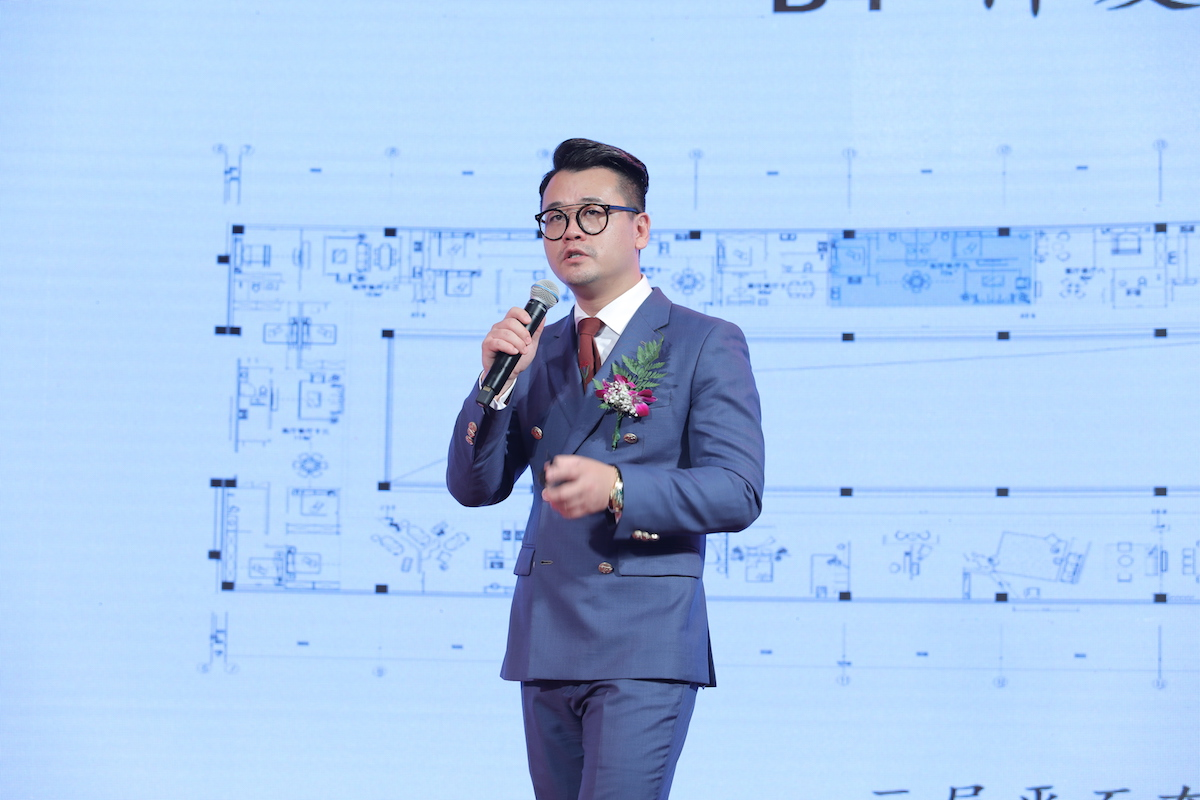庆典中最重要的环节是壹号馨家首席设计官吴巍向嘉宾阐释壹号馨家的品牌成立初衷和设计理念主张。吴巍讲到，之前我曾做了多年顶级豪宅的私人订制设计，但我发现这样的设计只能服务极少数的消费者，并不能服务到大多数的老百姓。基于这样的思考，我们总结了多年的设计经验，并整合了行业上下游最佳的供应链，向消费者呈现了我们在壹号馨家家居体验馆里的12套各具风格的样板间。壹号馨家希望通过这样“所见即所得”的服务，把家装变成一种产品研发的过程。让消费者购买家装服务就如同购买一件完整的商品一样，在消费的时候就可以看到最终呈现出来的品质，以及需要花费的整体时间和全部预算，全面改变传统家装模式，进而解决长期困扰在家装行业中装修效果不可控、工程造价不可控、工期不可控、最终装修品质不可控这四大痛点。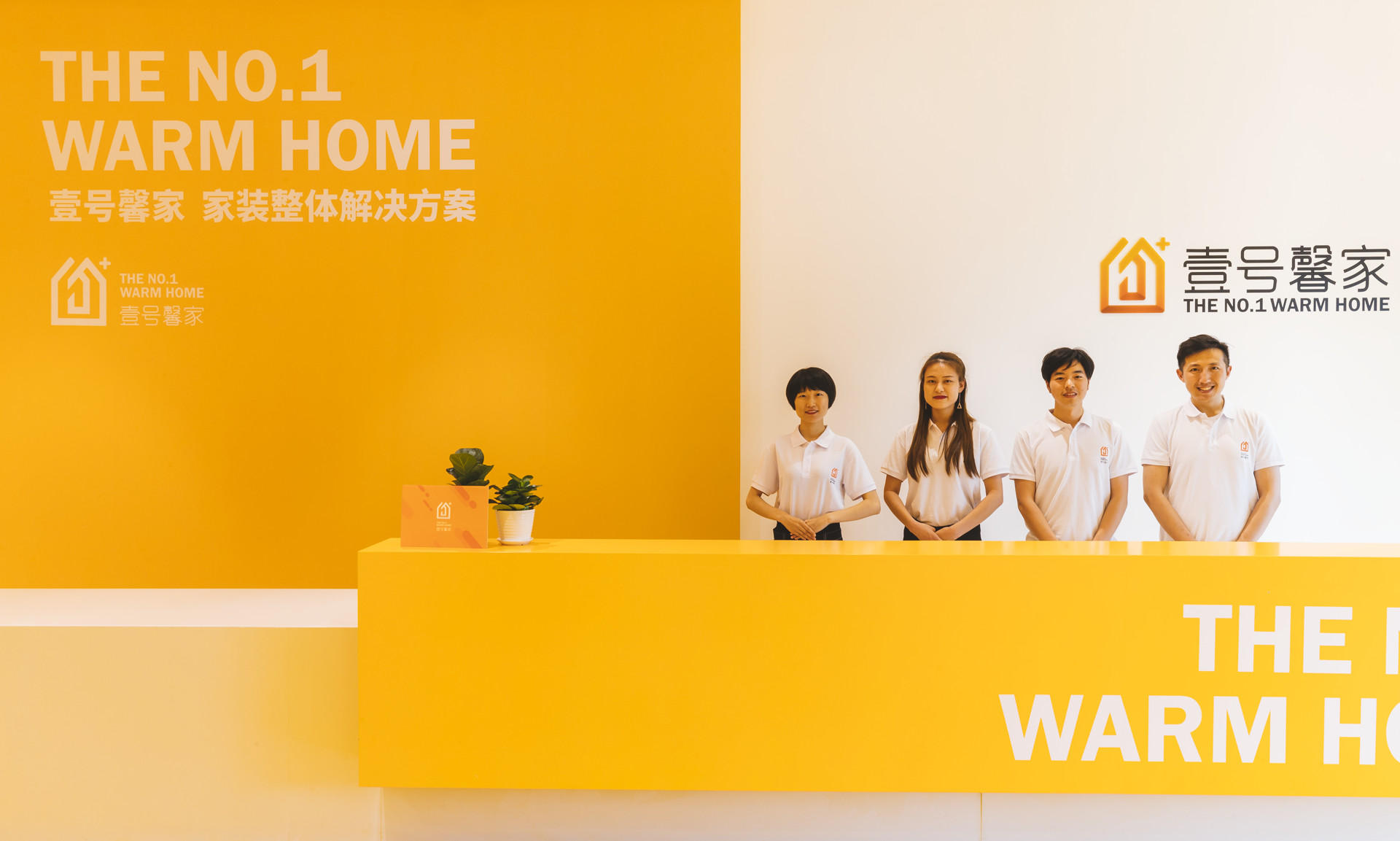随后，来自壹号馨家的监理、工程、设计、客服、供应商五大团队依次进行宣誓，表明了为京城家装消费者提供高品质产品与服务的决心和信心。11点18分，壹号馨家各位股东共同为壹号馨家家装体验馆的开幕剪彩，伴随着铿锵有力的锣鼓声与喝彩声，壹号馨家家装体验馆开业仪式圆满完成，壹号馨家家装体验馆正式开业！

全新一站式“全打包”家装服务体验装修再也不必东奔西跑

近年来，房地产市场雨后春笋般的蓬勃发展，让“居住”成为了关乎民生的社会问题，也直接带动了与居住相关的家装行业的快速发展。同时个性化、环保、生活美学等元素，也被越来越多的消费者关注和认可。另外，不断加快的生活节奏，也让消费者更倾向于寻找一种一站购齐、高品质、高颜值、高透明、高性价比的家装服务。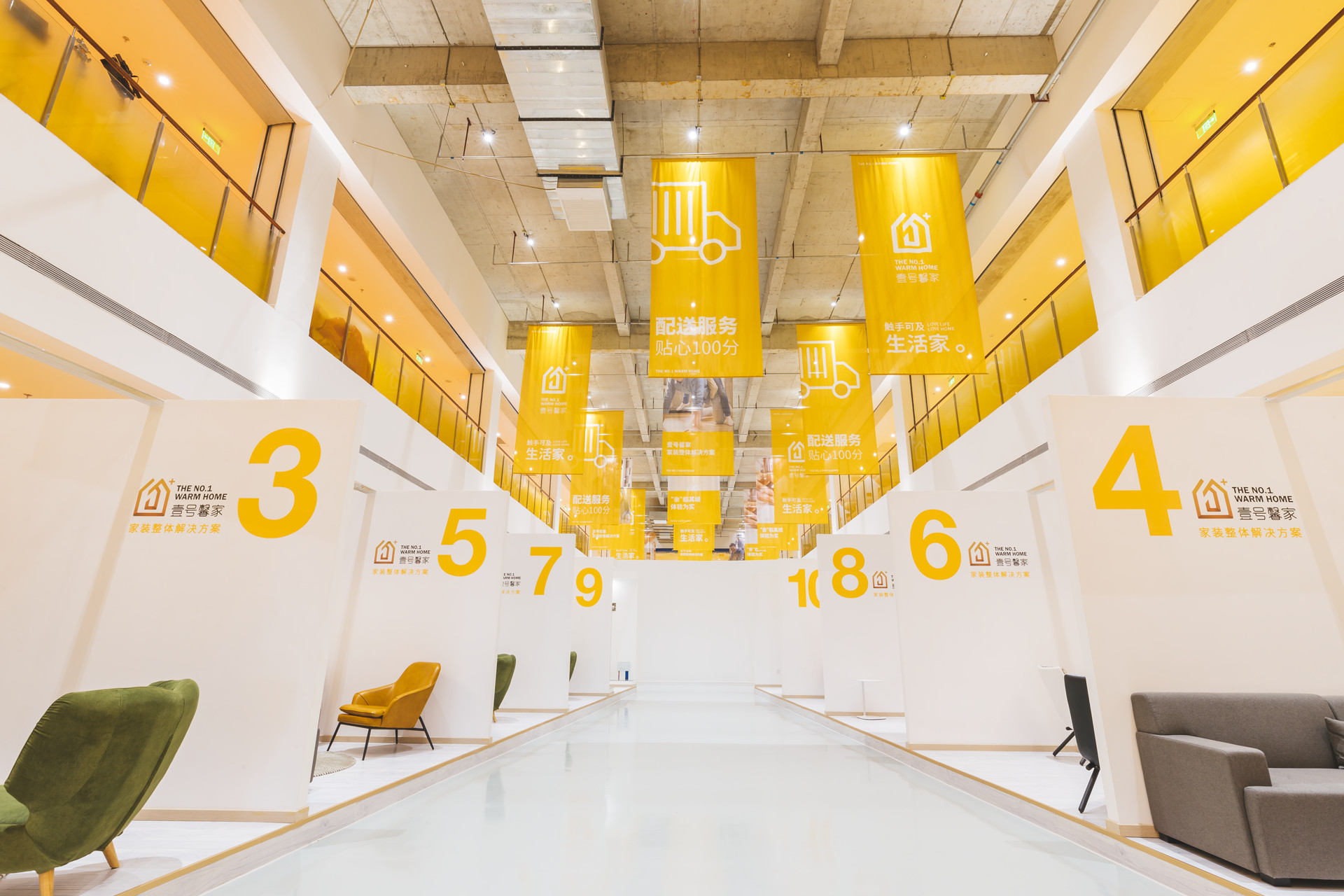作为全京城规模最大的一站式全透明家装服务体验品牌，在硬实力的全面调控下，壹号馨家不仅能为消费者提供专业时尚的家居整体规划和精心设计，营造全新的家居体验，更通过对整个家装行业产业链的全面整合，真正做到了家装行业中集设计、装修、建材、室内配套为一体的“一站式”服务，为消费者提供包含知名品牌建材、整体精细施工、大牌家电、精美家具、豪华软装在内的“全打包”式家装服务。真正让每一位业主省心、放心，不再东奔西跑，带来更加高效、实用、合理、美观、省钱的家装新体验。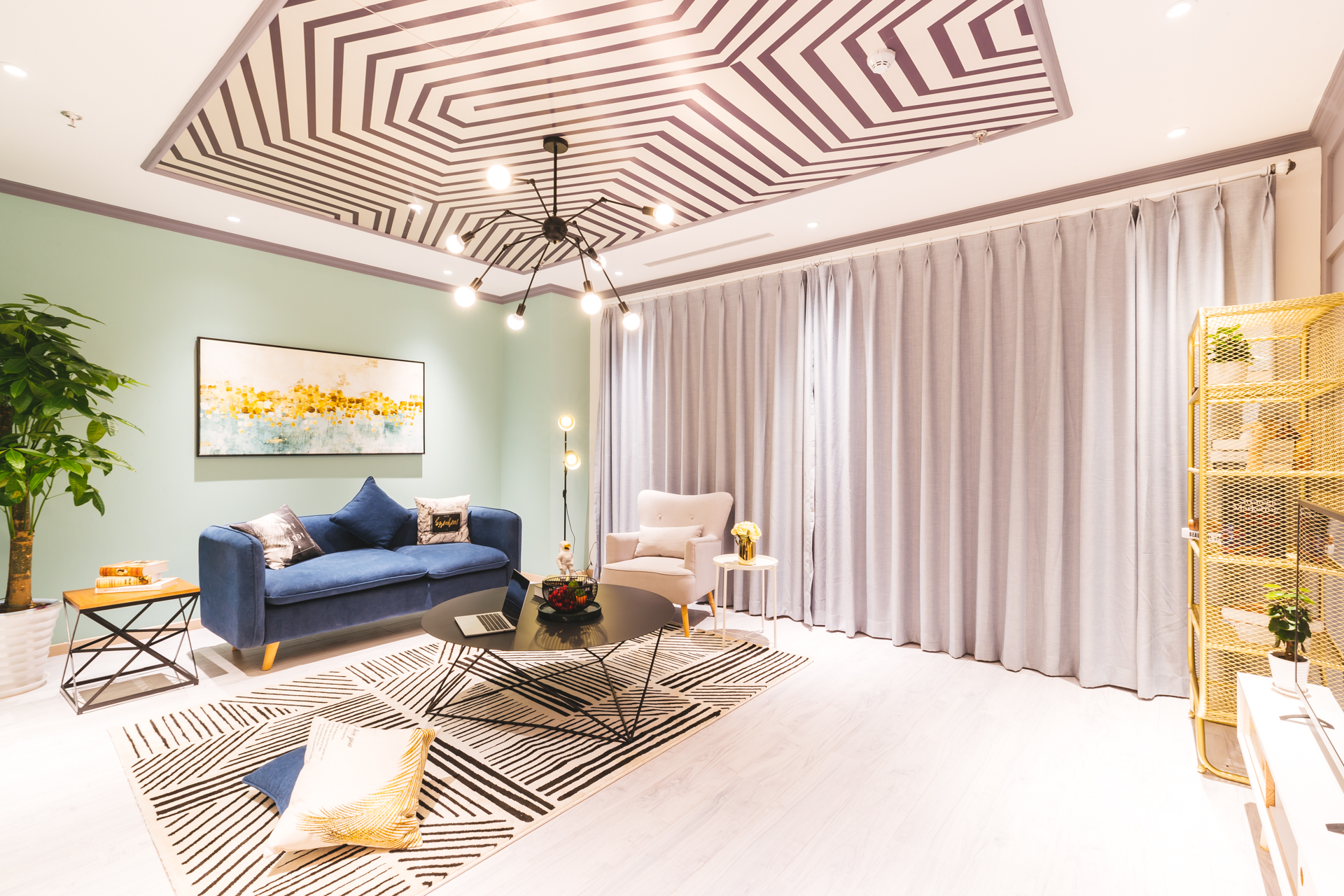在开业期间，壹号馨家还推出了“来就全包，来就送”的优惠政策与福利。届时，到店消费者不仅可以选择享受999元/平米特价样板间“豪华全包，无忧拎包入住”的礼遇，更有机会享受每平米减100元装修款，最高可省1万元的优惠。同时，消费者成功签单还可获赠4重豪礼：17件精美家具，6件大牌家电，6种地板、10种瓷砖随意搭，更有设计费、监理费全免全送。优惠绝对超值，让消费者真正全无忧入住。

`声明：本文由入驻焦点开放平台的作者撰写，除焦点官方账号外，观点仅代表作者本人，不代表焦点立场错误信息举报电话： 400-099-0099，邮箱：jubao@vip.sohu.com，或点此进行意见反馈，或点此进行举报投诉。`A B C D E F G H J K L M N P Q R S T W X Y Z
A - B - C - D - E
• A
• 鞍山
• 安庆
• 安阳
• 安顺
• 安康
• 澳门
• B
• 北京
• 保定
• 包头
• 巴彦淖尔
• 本溪
• 蚌埠
• 亳州
• 滨州
• 北海
• 百色
• 巴中
• 毕节
• 保山
• 宝鸡
• 白银
• 巴州
• C
• 承德
• 沧州
• 长治
• 赤峰
• 朝阳
• 长春
• 常州
• 滁州
• 池州
• 长沙
• 常德
• 郴州
• 潮州
• 崇左
• 重庆
• 成都
• 楚雄
• 昌都
• 慈溪
• 常熟
• D
• 大同
• 大连
• 丹东
• 大庆
• 东营
• 德州
• 东莞
• 德阳
• 达州
• 大理
• 德宏
• 定西
• 儋州
• 东平
• E
• 鄂尔多斯
• 鄂州
• 恩施
F - G - H - I - J
• F
• 抚顺
• 阜新
• 阜阳
• 福州
• 抚州
• 佛山
• 防城港
• G
• 赣州
• 广州
• 桂林
• 贵港
• 广元
• 广安
• 贵阳
• 固原
• H
• 邯郸
• 衡水
• 呼和浩特
• 呼伦贝尔
• 葫芦岛
• 哈尔滨
• 黑河
• 淮安
• 杭州
• 湖州
• 合肥
• 淮南
• 淮北
• 黄山
• 菏泽
• 鹤壁
• 黄石
• 黄冈
• 衡阳
• 怀化
• 惠州
• 河源
• 贺州
• 河池
• 海口
• 红河
• 汉中
• 海东
• 怀来
• I
• J
• 晋中
• 锦州
• 吉林
• 鸡西
• 佳木斯
• 嘉兴
• 金华
• 景德镇
• 九江
• 吉安
• 济南
• 济宁
• 焦作
• 荆门
• 荆州
• 江门
• 揭阳
• 金昌
• 酒泉
• 嘉峪关
K - L - M - N - P
• K
• 开封
• 昆明
• 昆山
• L
• 廊坊
• 临汾
• 辽阳
• 连云港
• 丽水
• 六安
• 龙岩
• 莱芜
• 临沂
• 聊城
• 洛阳
• 漯河
• 娄底
• 柳州
• 来宾
• 泸州
• 乐山
• 六盘水
• 丽江
• 临沧
• 拉萨
• 林芝
• 兰州
• 陇南
• M
• 牡丹江
• 马鞍山
• 茂名
• 梅州
• 绵阳
• 眉山
• N
• 南京
• 南通
• 宁波
• 南平
• 宁德
• 南昌
• 南阳
• 南宁
• 内江
• 南充
• P
• 盘锦
• 莆田
• 平顶山
• 濮阳
• 攀枝花
• 普洱
• 平凉
Q - R - S - T - W
• Q
• 秦皇岛
• 齐齐哈尔
• 衢州
• 泉州
• 青岛
• 清远
• 钦州
• 黔南
• 曲靖
• 庆阳
• R
• 日照
• 日喀则
• S
• 石家庄
• 沈阳
• 双鸭山
• 绥化
• 上海
• 苏州
• 宿迁
• 绍兴
• 宿州
• 三明
• 上饶
• 三门峡
• 商丘
• 十堰
• 随州
• 邵阳
• 韶关
• 深圳
• 汕头
• 汕尾
• 三亚
• 三沙
• 遂宁
• 山南
• 商洛
• 石嘴山
• T
• 天津
• 唐山
• 太原
• 通辽
• 铁岭
• 泰州
• 台州
• 铜陵
• 泰安
• 铜仁
• 铜川
• 天水
• 天门
• W
• 乌海
• 乌兰察布
• 无锡
• 温州
• 芜湖
• 潍坊
• 威海
• 武汉
• 梧州
• 渭南
• 武威
• 吴忠
• 乌鲁木齐
X - Y - Z
• X
• 邢台
• 徐州
• 宣城
• 厦门
• 新乡
• 许昌
• 信阳
• 襄阳
• 孝感
• 咸宁
• 湘潭
• 湘西
• 西双版纳
• 西安
• 咸阳
• 西宁
• 仙桃
• 西昌
• Y
• 运城
• 营口
• 盐城
• 扬州
• 鹰潭
• 宜春
• 烟台
• 宜昌
• 岳阳
• 益阳
• 永州
• 阳江
• 云浮
• 玉林
• 宜宾
• 雅安
• 玉溪
• 延安
• 榆林
• 银川
• Z
• 张家口
• 镇江
• 舟山
• 漳州
• 淄博
• 枣庄
• 郑州
• 周口
• 驻马店
• 株洲
• 张家界
• 珠海
• 湛江
• 肇庆
• 中山
• 自贡
• 资阳
• 遵义
• 昭通
• 张掖
• 中卫

1室1厅1厨1卫1阳台

1
2
3
4
5

0
1
2

1

1

0
1
2
3报名成功，资料已提交审核A B C D E F G H J K L M N P Q R S T W X Y Z
A - B - C - D - E
• A
• 鞍山
• 安庆
• 安阳
• 安顺
• 安康
• 澳门
• B
• 北京
• 保定
• 包头
• 巴彦淖尔
• 本溪
• 蚌埠
• 亳州
• 滨州
• 北海
• 百色
• 巴中
• 毕节
• 保山
• 宝鸡
• 白银
• 巴州
• C
• 承德
• 沧州
• 长治
• 赤峰
• 朝阳
• 长春
• 常州
• 滁州
• 池州
• 长沙
• 常德
• 郴州
• 潮州
• 崇左
• 重庆
• 成都
• 楚雄
• 昌都
• 慈溪
• 常熟
• D
• 大同
• 大连
• 丹东
• 大庆
• 东营
• 德州
• 东莞
• 德阳
• 达州
• 大理
• 德宏
• 定西
• 儋州
• 东平
• E
• 鄂尔多斯
• 鄂州
• 恩施
F - G - H - I - J
• F
• 抚顺
• 阜新
• 阜阳
• 福州
• 抚州
• 佛山
• 防城港
• G
• 赣州
• 广州
• 桂林
• 贵港
• 广元
• 广安
• 贵阳
• 固原
• H
• 邯郸
• 衡水
• 呼和浩特
• 呼伦贝尔
• 葫芦岛
• 哈尔滨
• 黑河
• 淮安
• 杭州
• 湖州
• 合肥
• 淮南
• 淮北
• 黄山
• 菏泽
• 鹤壁
• 黄石
• 黄冈
• 衡阳
• 怀化
• 惠州
• 河源
• 贺州
• 河池
• 海口
• 红河
• 汉中
• 海东
• 怀来
• I
• J
• 晋中
• 锦州
• 吉林
• 鸡西
• 佳木斯
• 嘉兴
• 金华
• 景德镇
• 九江
• 吉安
• 济南
• 济宁
• 焦作
• 荆门
• 荆州
• 江门
• 揭阳
• 金昌
• 酒泉
• 嘉峪关
K - L - M - N - P
• K
• 开封
• 昆明
• 昆山
• L
• 廊坊
• 临汾
• 辽阳
• 连云港
• 丽水
• 六安
• 龙岩
• 莱芜
• 临沂
• 聊城
• 洛阳
• 漯河
• 娄底
• 柳州
• 来宾
• 泸州
• 乐山
• 六盘水
• 丽江
• 临沧
• 拉萨
• 林芝
• 兰州
• 陇南
• M
• 牡丹江
• 马鞍山
• 茂名
• 梅州
• 绵阳
• 眉山
• N
• 南京
• 南通
• 宁波
• 南平
• 宁德
• 南昌
• 南阳
• 南宁
• 内江
• 南充
• P
• 盘锦
• 莆田
• 平顶山
• 濮阳
• 攀枝花
• 普洱
• 平凉
Q - R - S - T - W
• Q
• 秦皇岛
• 齐齐哈尔
• 衢州
• 泉州
• 青岛
• 清远
• 钦州
• 黔南
• 曲靖
• 庆阳
• R
• 日照
• 日喀则
• S
• 石家庄
• 沈阳
• 双鸭山
• 绥化
• 上海
• 苏州
• 宿迁
• 绍兴
• 宿州
• 三明
• 上饶
• 三门峡
• 商丘
• 十堰
• 随州
• 邵阳
• 韶关
• 深圳
• 汕头
• 汕尾
• 三亚
• 三沙
• 遂宁
• 山南
• 商洛
• 石嘴山
• T
• 天津
• 唐山
• 太原
• 通辽
• 铁岭
• 泰州
• 台州
• 铜陵
• 泰安
• 铜仁
• 铜川
• 天水
• 天门
• W
• 乌海
• 乌兰察布
• 无锡
• 温州
• 芜湖
• 潍坊
• 威海
• 武汉
• 梧州
• 渭南
• 武威
• 吴忠
• 乌鲁木齐
X - Y - Z
• X
• 邢台
• 徐州
• 宣城
• 厦门
• 新乡
• 许昌
• 信阳
• 襄阳
• 孝感
• 咸宁
• 湘潭
• 湘西
• 西双版纳
• 西安
• 咸阳
• 西宁
• 仙桃
• 西昌
• Y
• 运城
• 营口
• 盐城
• 扬州
• 鹰潭
• 宜春
• 烟台
• 宜昌
• 岳阳
• 益阳
• 永州
• 阳江
• 云浮
• 玉林
• 宜宾
• 雅安
• 玉溪
• 延安
• 榆林
• 银川
• Z
• 张家口
• 镇江
• 舟山
• 漳州
• 淄博
• 枣庄
• 郑州
• 周口
• 驻马店
• 株洲
• 张家界
• 珠海
• 湛江
• 肇庆
• 中山
• 自贡
• 资阳
• 遵义
• 昭通
• 张掖
• 中卫• 手机• 分享
• 设计
免费设计
• 计算器
装修计算器
• 入驻
合作入驻
• 联系
联系我们
• 置顶
返回顶部